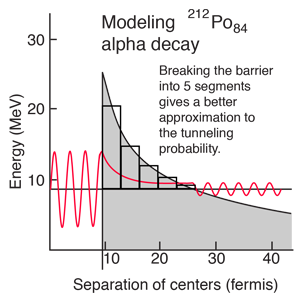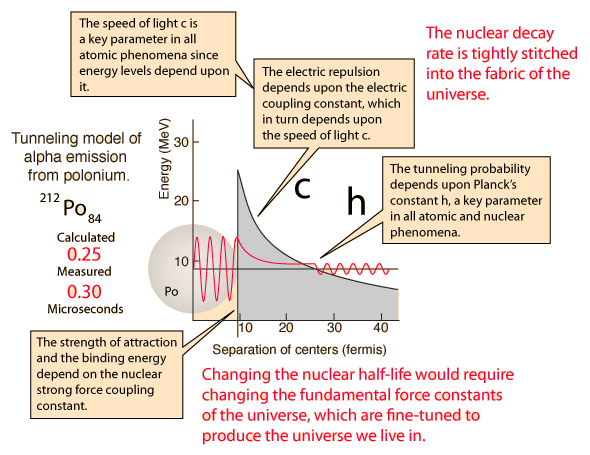# Modeling Polonium-212 Alpha Half-lifeThe incredible range of alpha decay half-lives can be modeled with quantum mechanical tunneling. The illustration represents the barrier faced by an alpha particle in polonium-212, which emits an 8.78 MeV alpha particle with a half-life of 0.3 microseconds. The following characteristics of the nuclear environment can be calculated from a basic model of the nucleus:

 Separation of centers of alpha and nucleus at edge of barrier 9.1 fm Height of barrier 26.4 MeV Radius at which barrier drops to alpha energy 26.9 fm Width of barrier seen by alpha 17.9 fm Alpha's frequency of hitting the barrier 1.1 x 1021/s
 Details of calculation Different isotopes

The shape of the barrier must obviously be taken into account since it drops rapidly. But it is instructive to calculate the half-life for a rectangular barrier of that height and width. Using the barrier penetration calculation gives a half-life of 1.5 x 107 seconds, about 13 orders of magnitude longer than the observed half-life. This gives some insight into the large range of alpha half-lives; the tunneling probability depends very strongly on the nature of the barrier. The probability depends exponentially on the height and width, and in this case the height is dropping like 1/r with distance from the nucleus.A better tunneling probability can be obtained by breaking the barrier into segments and multiplying the successive tunneling probabilities according to the basic rules for combining probabilities. The calculated tunneling probabilities for the segments are shown in the table.

 Segment height Probability of tunneling 21.9 1.20 x10-5 16.4 1.74 x 10-4 13.2 1.43 x 10-3 11.0 .98 x 10-2 9.4 .85 x 10-1 Product ofprobabilities 2.47 x 10-15
 Details of calculation

When this tunneling probability is used to calculate the half-life, a value of 0.25 microseconds is obtained. This is surprisingly close to the measured value of 0.3 microseconds, considering that an incorrect barrier can take you off many orders of magnitude. It should be admitted that the same calculation for other nuclei does not yield such close agreement, but this example shows that the observed half-lives are within the range which can be calculated from quantum mechanical tunneling.

 What could you do to change the half-life?
Index

Alpha Decay Concepts

References
Rohlf
Sec 7-4

Eisberg & Resnick
Sec 16-2

 HyperPhysics***** Nuclear R Nave
Go Back

# What could you do to change the polonium half-life?

For the modeling of the alpha decay half-life of polonium-212 with the tunneling probability, a value of 0.25 microseconds was obtained. This is surprisingly close to the measured value of 0.3 microseconds, considering that an incorrect barrier can take you off many orders of magnitude. It suggests that the major factors affecting such alpha decay have been accounted for in a reasonable manner.

What could you do to change the polonium half-life? The answer is that there is no way to experimentally change that parameter because it ultimately depends upon the values of fundamental physical constants. The illustration below points out that the characteristics which affect the barrier and the tunneling probability are:

1. The nuclear radius and the depth of the nuclear potential well, which depend upon the coupling strength for the nuclear strong force.
2. The electric repulsion, which depends upon Coulomb's constant, which in turn depends upon the speed of light c.
3. The tunneling probability, which depends upon Planck's constant.The forces inside the nucleus are balanced on a razor's edge. The constants that determine nuclear decay rate are stitched tightly into the fabric of the cosmos. The decay rate can't be changed without changing essentially all of chemistry and physics.Index

Alpha Decay Concepts

References
Rohlf
Sec 7-4

Eisberg & Resnick
Sec 16-2

 HyperPhysics***** Nuclear R Nave
Go Back